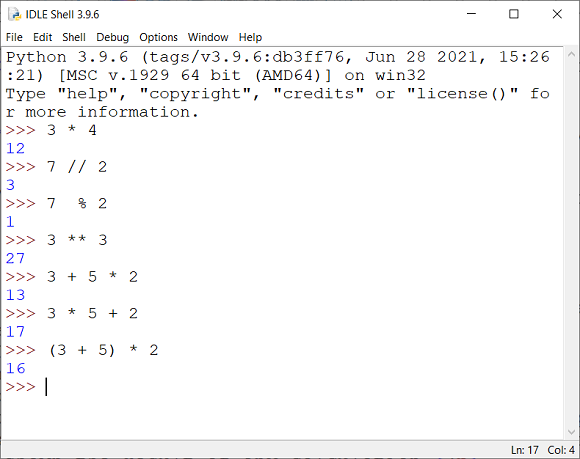# Python For GCSE Arithmetic Operators

The table shows the operators that are used for arithmetic in Python.

OperatorMeaningExampleExample Result
-Subtract7 - 34
*Multiply2 * 612
/Divide12 / 34
//Divide and ignore the remainder (floor division)10 // 33
**Raise to the power2 ** 38
%Give the remainder when the first number is divided by the second (modulus)7 % 21

In GCSE questions,the pseudocode will use DIV to indicate floor division. It will also use MOD to indicate the modulus (remainder) operator.

## Using The Python Shell

IDLE (Integrated Development & Learning Environment) is bundled with the default installation of Python. When we first launch IDLE, we see a Python Shell. This is a tool which can run our Python programs for us. It will also allow us to type Python statements, one a time, a see the results. This feature is called a REPL (Read Evaluate Print Loop). When we enter a statement, we will be shown the result of any calculation.

Most of the time, we will write our Python programs in blank files and, when we run them, the shell is loaded to run the program. Sometimes it can be useful to type lines into Python to test statements or to do something quickly.

You can test out the operators in the shell.If you study this screenshot carefully, you can spot a few things. Firstly, we can use the shell as a calculator, typing in arithmetic expressions and seeing the results. Secondly, we can see how the floor division, modulus and power operators work. Thirdly, we can see that the order of operations follows the BIDMAS rule and that brackets may be needed to ensure that some of our expressions are calculated in the correct order.